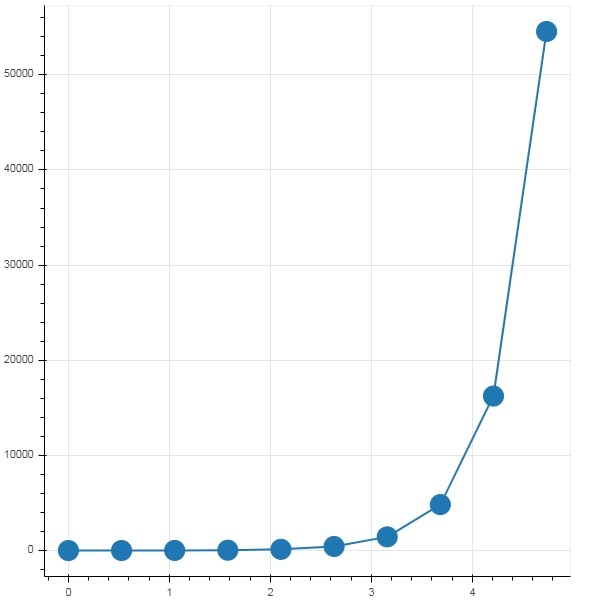# Bokeh - Pandas

In all the examples above, the data to be plotted has been provided in the form of Python lists or numpy arrays. It is also possible to provide the data source in the form of pandas DataFrame object.

DataFrame is a two-dimensional data structure. Columns in the dataframe can be of different data types. The Pandas library has functions to create dataframe from various sources such as CSV file, Excel worksheet, SQL table, etc.

For the purpose of following example, we are using a CSV file consisting of two columns representing a number x and 10x. The test.csv file is as below −

```x,pow
0.0,1.0
0.5263157894736842,3.3598182862837818
1.0526315789473684,11.28837891684689
1.5789473684210527,37.926901907322495
2.1052631578947367,127.42749857031335
2.631578947368421,428.1332398719391
3.1578947368421053,1438.449888287663
3.6842105263157894,4832.930238571752
4.2105263157894735,16237.76739188721
4.7368421052631575,54555.947811685146
```

We shall read this file in a dataframe object using read_csv() function in pandas.

```import pandas as pd
print (df)
```

The dataframe appears as below −

```x        pow
0 0.000000 1.000000
1 0.526316 3.359818
2 1.052632 11.288379
3 1.578947 37.926902
4 2.105263 127.427499
5 2.631579 428.133240
6 3.157895 1438.449888
7 3.684211 4832.930239
8 4.210526 16237.767392
9 4.736842 54555.947812
```

The ‘x’ and ‘pow’ columns are used as data series for line glyph in bokeh plot figure.

```from bokeh.plotting import figure, output_file, show
p = figure()
x = df['x']
y = df['pow']
p.line(x,y,line_width = 2)
p.circle(x, y,size = 20)
show(p)
```

### Output